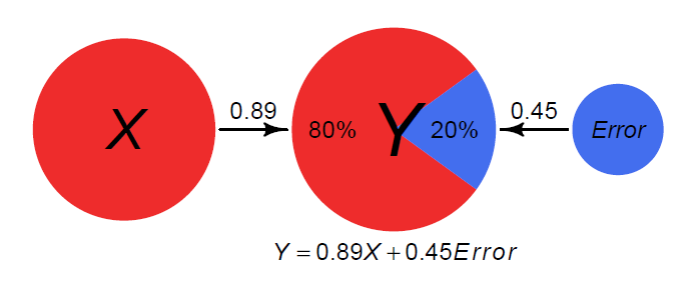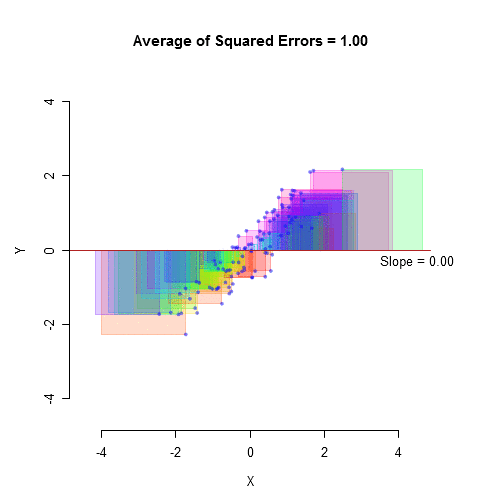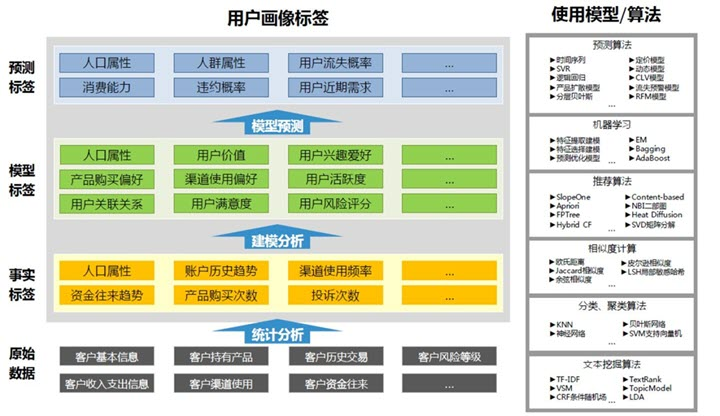# 回归模型评估指标

• explained_variance_score(y_true, y_pred) Explained variance regression score function
• mean_absolute_error(y_true, y_pred) Mean absolute error regression loss
• mean_squared_error(y_true, y_pred[, …]) Mean squared error regression loss
• mean_squared_log_error(y_true, y_pred) Mean squared logarithmic error regression loss
• median_absolute_error(y_true, y_pred) Median absolute error regression loss
• r2_score(y_true, y_pred[, …]) R^2 (coefficient of determination) regression score function.

## 可解释方差Explained Variance Score

$$Explained\ Variance = 1 – \frac{Var(y – f)}{Var(y)}$$## 平均绝对误差 Mean Absolute Error(MAE)

MAE用来描述预测值和真实值的差值。数值越小越好。假设$y_i$是真实值，$f_i$是相对应的预测值，则n个样本的MAE可由下式出给：

$$MAE = \frac{1}{n} \sum_{i=1}^{n}|f_i-y_i|$$

MAE优缺点：虽然平均绝对误差能够获得一个评价值，但是你并不知道这个值代表模型拟合是优还是劣，只有通过对比才能达到效果。

## 均方误差 Mean Squared Error(MSE)

Mean Squared Error也称为Mean Squared Deviation(MSD)，计算的是预测值和实际值的平方误差。同样，数值越小越好。

$$MSE = \frac{1}{n} \sum_{i=1}^{n}(f_i – y_i)^2$$

## 均方根误差 Root Mean Squared Error（RMSE）

$$RMSE = \sqrt{\frac{1}{n} \sum_{i=1}^{n}(f_i – y_i)^2}$$

RMSE与MAE对比：RMSE相当于L2范数，MAE相当于L1范数。次数越高，计算结果就越与较大的值有关，而忽略较小的值，所以这就是为什么RMSE针对异常值更敏感的原因（即有一个预测值与真实值相差很大，那么RMSE就会很大）。

## 均值平方对数误差 Mean Squared Log Error(MSLE)

$$\text{MSLE}(y, \hat{y}) = \frac{1}{n_\text{samples}} \sum_{i=0}^{n_\text{samples} – 1} (\log_e (1 + y_i) – \log_e (1 + \hat{y}_i) )^2$$

## 中位数绝对误差 Median Absolute Error（MedianAE）

$$\text{MedAE}(y, \hat{y}) = \text{median}(\mid y_1 – \hat{y}_1 \mid, \ldots, \mid y_n – \hat{y}_n \mid)$$

## R-平方 $R^2$ Score

$R^2$ Score又称为the coefficient of determination。判断的是预测模型和真实数据的拟合程度，最佳值为1，同时可为负值。

$$R^2 = 1 – \frac{\sum_{i=1}^{n}(y_i – f_i)^2}{\sum_{i=1}^{n}(y_i – \overline{y})^2}$$##### 抖音推荐机制与算法的研究##### 张小龙、张一鸣谁能笑傲江湖